Honza

Honza is 13 years old and Peter 21 years old. After how many years are their ages will be at ratio 7: 9?

Result

x =  15

Solution:

9*(13+x) = 7*(21+x)

2x = 30

x = 15

Calculated by our simple equation calculator.

Leave us a comment of this math problem and its solution (i.e. if it is still somewhat unclear...):Be the first to comment!To solve this verbal math problem are needed these knowledge from mathematics:

Need help calculate sum, simplify or multiply fractions? Try our fraction calculator. Do you have a linear equation or system of equations and looking for its solution? Or do you have quadratic equation?

Next similar math problems:

1. MidnightHow many hours are, if the time that elapsed since 8:00 is 2/5 of the time that will past till midnight?
2. Three monksThree medieval monks has task to copy 600 pages of the Bible. One rewrites in three days 1 page, second in 2 days 3 pages and a third in 4 days 2 sides. Calculate for how many days and what day the monks will have copied whole Bible when they begin Wednesd
3. Two typists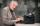There are two typists who are rewriting the material 814 pages. First can it handle rewrite yourself for 24 days; the second 12 days. First typist wrote material yourself 4 days rest rewrites yourself second typist. How many days will it take rewriting alt
4. School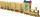School attend 792 children, boys and girls ratio is 4:5. How many more girls go to school (than boys)?
5. There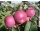There are 32 trees of three species in the orchard. 25% cherries, three eighty pears, others plums. How many plums are in the orchard?
6. PearsThere were pears in the basket, I took two-fifths of them, and left six in the basket. How many pears did I take?
7. Three peopleThree people start doing a work at a same time. The first worked only 2 hours. The second ended 3 hours before the end. On an individual basis, it would take the first time to do the work 10 hours, second 12 hours and 15 hours third. How many hours did it.
8. Ratio 11Simplify this ratio 10 : 1/4
9. New ratio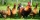The ratio of ducks and chicken in our yard is 2 : 3. The total number of ducks and chickens together is 30. Mother gave 3 of the chickens to our neighbor. What is the new ratio now?
10. Percentage 1052 shorts and missed 13. Calculate percentage
11. Unknown number 23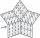Find 2/3 of unknown number, which is two thirds of the 99.
12. Equation with mixed fractions2 3/5 of 1430+? = 1900. How to do this problem
13. One thirdIf 3/5 is 360, how much is 1/3?
14. EqnSolve equation with fractions: 2x/3-50=40+x/4
15. Unknown numberI think the number - its sixth is 3 smaller than its third.
16. Eq-fracSolve the following equation with fractions: h + 1/3 =5/3
17. Simple equation 5Solve equation with fractions: X × 3/8 = 1/2